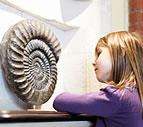×

# probability

1 ENTRIES FOUND:
probability /ˌprɑːbəˈbɪləti/ noun
plural probabilities
probability
/ˌprɑːbəˈbɪləti/
noun
plural probabilities
Learner's definition of PROBABILITY
: the chance that something will happen
[count]
[noncount]
[singular] : something that has a chance of happening
: a measure of how often a particular event will happen if something (such as tossing a coin) is done repeatedly
[count]
[noncount]

## in all probability

: almost certainly : very likely
ASK THE EDITORLEARNER'S QUIZZES
LEARNER'S WORD OF THE DAY